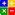# Ratio - Altman Z ScoreALTMAN Z-SCORE:Online Z-Score Calculator

Using Multiple Discriminant Analysis Edward Altman combined a set of 5 financial ratios to come up with the Altman Z-Score. This score uses statistical techniques to predict a company's probability of failure using the following 8 variables from a company's financial statements:
{For Z Score the following 8 inputs are essential: {Current Assets; Current Liabilities; Total Liabilities; EBIT; Total Assets; Net Sales; Retained Earnings; Market Value of Equity}

The 5 financial ratios in the Altman Z-Score and their respective weight factor is as follows:

 RATIO WEIGHTAGE A EBIT/Total Assets x. 3.3 -4 to +8.0 B Net Sales /Total Assets x 0.999 -4 to +8.0 C Market Value of Equity / Total Liabilities x 0.6 -4 to +8.0 D Working Capital/Total Assets x 1.2 -4 to +8.0 E Retained Earnings /Total Assets x1.4 -4 to +8.0

These ratios are multiplied by the weightage as above, and the results are added together.
Z-Score = A x 3.3 + B x 0.99 + C x 0.6 + D x 1.2 + E x 1.4

The Interpretation of Z Score:

Z-SCORE ABOVE 3.0 -The company is safe based on these financial figures only.
Z-SCORE BETWEEN 2.7 and 2.99 - On Alert. This zone is an area where one should exercise caution.
Z-SCORE BETWEEN 1.8 and 2.7 - Good chances of the company going bankrupt within 2 years of operations from the date of financial figures given.
Z-SCORE BELOW 1.80- Probability of Financial embarassment is very high.